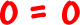The last freaky thing that can happen is that all three planes intersect in a line...So, there are a ton of points along that line that work in all three equations.  An infinite number!

When you are doing the elimination method, you'll be working through...  adding those equations together...  and BOOM!

You'll get this:Yeah, it's a true statement...  But not very helpful.

When this happens, you'll know that the planes all intersect in a line -- and that line IS the answer!  In some harder classes, you'll be shown how to find the equation of that line.  For now, write something like this:

"The three planes intersect in a line.
So, there are an infinite number of solutions."